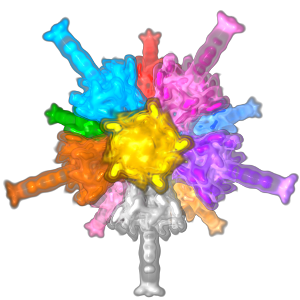#### Symmetry in scalar fields.

Dilip Mathew Thomas

#### Abstract

Scalar fields are used to represent physical quantities measured over a domain of interest. Study of symmetric or repeating patterns in scalar fields is important in scientific data analysis because it gives deep insights into the properties of the underlying phenomenon.

This thesis proposes three methods to detect symmetry in scalar fields. The first method models symmetry detection as a subtree matching problem in the contour tree, which is a topological graph abstraction of the scalar field. The contour tree induces a hierarchical segmentation of features at different scales a nd hence this method can detect symmetry at different scales. The second method identifies symmetry by comparing distances between extrema from each symmetric region. The distance is computed robustly using a topological abstraction called the extremum graph. Hence, this method can detect symmetry even in the presence of significant noise. The above methods compare pairs of regions to identify symmetry instead of grouping the entire set of symmetric regions as a cluster. This motivates the third method which uses a clustering analysis for symmetry detection. In this method, the contours of a scalar field are mapped to points in a high-dimensional descriptor space such that points corresponding to similar contours lie in close proximity to each other. Symmetry is identified by clustering the points in the descriptor space.

We show through experiments on real world data sets that these methods are robust in the presence of noise and can detect symmetry under different types of transformations. Extraction of symmetry information helps users in visualization and data analysis. We design novel applications that use symmetry information to enhance visualization of scalar field data and to facilitate their exploration.

[PDF]